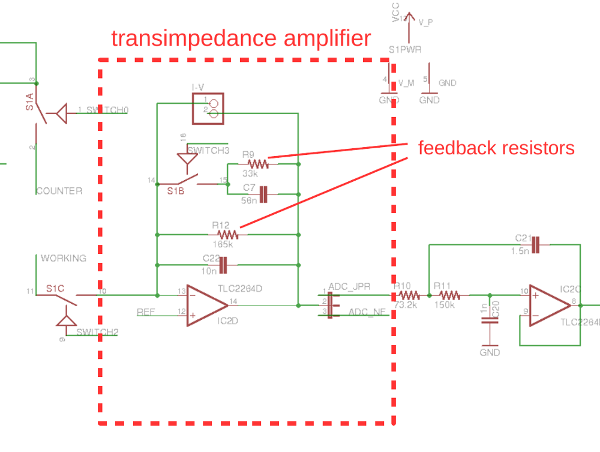# Dye sensitized solar cell analysis browsing

• Is it possible to use the Cheapstat in a two electrode mode to determine the I-V curve of a dye sensitized solar cell. I am new to this and if anyone has some suggestions it would be helpful.

• Maybe - what sort of voltage and current range would you need for this? With the stock feedback resistors in the transimpedance amplifier the current ranges for the cheapstat are +/10uA and +/-50uA. I'm not very familiar with this application, but looking up some sample I-V curves online I see currents in the 100-600uA range which would probably be too high for the cheapstat. It is possible to modify the current range by changing the resistors for the transimpedance amplifier. This would involve replacing one or more surface mount resistors.

• Thanks for the information. I probably need a 100micro amp range. But if a resistor change can do it, it might be worth a try.

• On the Cheapstat schematic there is a jumper labeled I-V Converter. Would placing a resistance here (parallel to the 165k) increase the current limit of the TIA? Any problems with this ? eg adding 165k would give a 100micro amp limit. Or am I wrong here.

• @tmccaffr I think this would work. See the image below.Resistors R9 and R12 set the current ranges for the transimpedance amplifier. Depending on the setting of switch3 (in firmware) you have either R12 alone for the transimpedance amplifier or R9 and R12 in parallel. The I-V jumper, which you mention, is in correct position. A resistor you put across this jumper will be in parallel with the other feedback resistors - so by adding a resistor you could reduce the sensitivity of the amplifier.

• Is it possible to up the range to around 0 to 70mA? If so, what resistance would I use?

• @tmccaffr Hi Will,

I solved the original question I had by adding a 160 ohm resistor across the bridge as suggested in a previous reply. Before doing this though I was getting current readings of up to around 1600 on the 0-50uA setting. Can I confirm what units this is? If the max is 50uA I am not sure what 1600 means.

Thanks,

Daniel

• @droxby

The values returned are the raw integers read from the ADC (analog to digital converter) on the output of the transimpedance amplifier (current to voltage converter). You will need to convert these integer values to current measurements e.g. uA. While it is possible to do a first principles calculation using the values of the feedback resistor in the transimpedance amplifier it is generally better to just do a simple calibration using a dummy cell consisting of a single resistor (of known value R).

The basic idea is to connect the counter and reference electrodes to one side of the resistor and the working electrode to the other side. You then want to sweep the voltages through a range of values - e.g. via a cyclic voltammetry test. Because the resistance R is known for each output voltage v(t) in the test you can calculate the current i(t) = v(t)/R through the resistor. At the same time you have the measured ADC integer values n(t) corresponding to this current. To get the calibration you can just perform a linear fit between the ADC integers n(t) and the know current values i(t).

When performing the calibration test make sure to select an appropriate resistor. You want to generate currents which span the input range (for current), but don't go outside of it. The transimpedance amplifier can't generate voltages higher then 3.3V or lower than 0V - so if you go outside of the current range you will get clipping (or saturation) of the output which will show up as a flat spot in your calibration data.